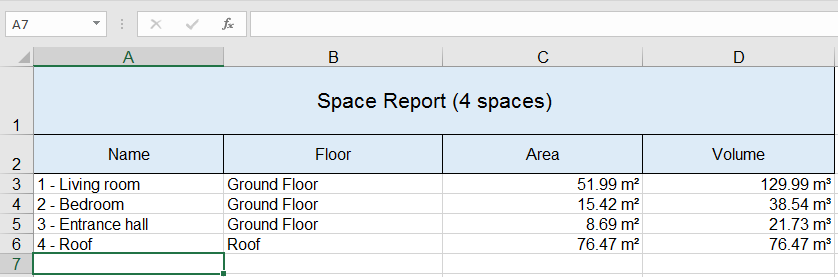﻿ Excel Space Report from IFC

# Excel Space Report from IFC

This example will show you some of the concepts necessary to read data from IFC file. It uses IFC4 interfaces which will work both for IFC2x3 and IFC4 models. To create Excel files we use NPOI. You will just need xBIM Essentials for this example because we are not going to use geometry here. All the code including sample data is available here.

The result from this example will look like this:You will need these `using` statements:

``````using NPOI.SS.UserModel;
using NPOI.XSSF.UserModel;
using System.Diagnostics;
using System.IO;
using System.Linq;
using Xbim.Ifc;
using Xbim.Ifc4.Interfaces;
``````

The main function looks like this:

``````//initialize NPOI workbook from the template
var workbook = new XSSFWorkbook("template.xlsx");
var sheet = workbook.GetSheet("Spaces");

//Create nice numeric formats with units. Units would need a LOT MORE care in real world.
//We just know that our current model has space areas in square meters and space volumes in cubic meters
//Please note that the original data exported from Revit were wrong because volumes were 1000x bigger than they should be.
//Data were fixed using xBIM for this example.

var areaFormat = workbook.CreateDataFormat();
var areaFormatId = areaFormat.GetFormat("# ##0.00 [\$m²]");
var areaStyle = workbook.CreateCellStyle();
areaStyle.DataFormat = areaFormatId;

var volumeFormat = workbook.CreateDataFormat();
var volumeFormatId = volumeFormat.GetFormat("# ##0.00 [\$m³]");
var volumeStyle = workbook.CreateCellStyle();
volumeStyle.DataFormat = volumeFormatId;

//Open IFC model. We are not going to change anything in the model so we can leave editor credentials out.
using (var model = IfcStore.Open("SampleHouse.ifc"))
{
//Get all spaces in the model.
//We use ToList() here to avoid multiple enumeration with Count() and foreach(){}
var spaces = model.Instances.OfType<IIfcSpace>().ToList();
sheet.GetRow(0).GetCell(0)
.SetCellValue(\$"Space Report ({spaces.Count} spaces)");
foreach (var space in spaces)
{
//write report data
WriteSpaceRow(space, sheet, areaStyle, volumeStyle);
}
}

//save report
using (var stream = File.Create("spaces.xlsx"))
{
workbook.Write(stream);
stream.Close();
}

//see the result if you have some SW associated with the *.xlsx
Process.Start("spaces.xlsx");
``````

This code uses following functions to read the data from IFC and write a row for every space.

``````private static void WriteSpaceRow(IIfcSpace space, ISheet sheet, ICellStyle areaStyle, ICellStyle volumeStyle)
{
var row = sheet.CreateRow(sheet.LastRowNum + 1);

var name = space.Name;
row.CreateCell(0).SetCellValue(name);

var floor = GetFloor(space);
row.CreateCell(1).SetCellValue(floor?.Name);

var area = GetArea(space);
if (area != null)
{
var cell = row.CreateCell(2);
cell.CellStyle = areaStyle;

//there is no guarantee it is a number if it came from property and not from a quantity
if (area.UnderlyingSystemType == typeof(double))
cell.SetCellValue((double)(area.Value));
else
cell.SetCellValue(area.ToString());
}

var volume = GetVolume(space);
if (volume != null)
{
var cell = row.CreateCell(3);
cell.CellStyle = volumeStyle;

//there is no guarantee it is a number if it came from property and not from a quantity
if (volume.UnderlyingSystemType == typeof(double))
cell.SetCellValue((double)(volume.Value));
else
cell.SetCellValue(volume.ToString());
}
}
``````

To get both floor and properties or quantities you need to inspect objectified relations. For example to get the floor which contains the space you need to do this:

``````private static IIfcBuildingStorey GetFloor(IIfcSpace space)
{
return
//get all objectified relations which model decomposition by this space
space.Decomposes

//select decomposed objects (these might be either other space or building storey)
.Select(r => r.RelatingObject)

//get only storeys
.OfType<IIfcBuildingStorey>()

//get the first one
.FirstOrDefault();
}
``````

IFC contains data infrastructure to store any arbitrary data related to products and their types. This infrastructure is rather complex. Two main ways to store the data is as a quantity or as a property. Quantities are explicit about the type of value they contain where properties can contain many different data types as a value. For area and volume it is preferable to get the value from quantities if they are defined. Property is a fallback in this case.

``````private static IIfcValue GetArea(IIfcProduct product)
{
//try to get the value from quantities first
var area =
//get all relations which can define property and quantity sets
product.IsDefinedBy

//Search across all property and quantity sets.
//You might also want to search in a specific quantity set by name
.SelectMany(r => r.RelatingPropertyDefinition.PropertySetDefinitions)

//Only consider quantity sets in this case.
.OfType<IIfcElementQuantity>()

//Get all quantities from all quantity sets
.SelectMany(qset => qset.Quantities)

//We are only interested in areas
.OfType<IIfcQuantityArea>()

//We will take the first one. There might obviously be more than one area properties
//so you might want to check the name. But we will keep it simple for this example.
.FirstOrDefault()?
.AreaValue;

if (area != null)
return area;

//try to get the value from properties
return GetProperty(product, "Area");
}

private static IIfcValue GetVolume(IIfcProduct product)
{
var volume = product.IsDefinedBy
.SelectMany(r => r.RelatingPropertyDefinition.PropertySetDefinitions)
.OfType<IIfcElementQuantity>()
.SelectMany(qset => qset.Quantities)
.OfType<IIfcQuantityVolume>()
.FirstOrDefault()?.VolumeValue;
if (volume != null)
return volume;
return GetProperty(product, "Volume");
}
``````

It is probably more common to search for values in property sets so this is how you can do it.

``````private static IIfcValue GetProperty(IIfcProduct product, string name)
{
return
//get all relations which can define property and quantity sets
product.IsDefinedBy

//Search across all property and quantity sets. You might also want to search in a specific property set
.SelectMany(r => r.RelatingPropertyDefinition.PropertySetDefinitions)

//Only consider property sets in this case.
.OfType<IIfcPropertySet>()

//Get all properties from all property sets
.SelectMany(pset => pset.HasProperties)

//lets only consider single value properties. There are also enumerated properties,
//table properties, reference properties, complex properties and other
.OfType<IIfcPropertySingleValue>()

//lets make the name comparison more fuzzy. This might not be the best practise
.Where(p =>
string.Equals(p.Name, name, System.StringComparison.OrdinalIgnoreCase) ||
p.Name.ToString().ToLower().Contains(name.ToLower()))

//only take the first. In reality you should handle this more carefully.
.FirstOrDefault()?.NominalValue;
}
``````

Some of the companies using xBIM Toolkit: